## Rate of Change: Algebra

The rate of change, the rate of motion, the rate of a heartbeat. A chart on a piece of paper as boring as it may seem, can produce some pretty great numbers that directly relate to us human folk.

The question below may make you groan at first glance, but what happens if we use our imagination to picture the real-life bird it’s describing? Any better? Yes? No? Well, either way we must solve, so let’s get to it

## How do I answer this question?

It wants us to find the Rate of Change, specifically between 3 and 9 seconds. Let’s hi-light those two values on our given table:

## What is the Rate of Change?

To get the Rate of Change between these two values, we need to go back to the good ole’ Slope Formula and realize that the list of values is really a list of x and y coordinates.

Now let’s plug in the coordinate values (3, 6.26) and (9,3.41) into our slope formula:

Extra Tip! Notice that we added the labels feet/second to our answer.  Why does this make sense?? The question tells us that P(t) represents feet and that t is equal to seconds.  Another way to look at this question when applying it to the slope formula is to realize that we are finding the change of feet divided by the change of seconds.                                                          ____________________________________________________________________________________

Still got questions?  Let me know in the comments and as always happy calculating!:)

Looking for the next step? Learn how to graph equation of a line, y=mx+b here!

## How to Factor Quadratic Equations: Algebra

In this post, we are going to dive deep into how to factor Quadratic equations! There are so many different methods to choose from including GCF, Product/Sum, DOTS, and the Quadratic Formula.  Here we will go step by step into each method on how to factor quadratic equations, each with their own set of practice questions. For a review on how to factor by grouping, check out this post here and  happy calculating! 🙂

Why factor in the first place, you may say? We want to manipulate the equation until we solve for x.  Solving for x is our main goal, and factoring allows us to do that.  Now let’s get to the good stuff!## Greatest Common Factor (GCF):

The greatest common factor is the highest possible number that can be divided out from an equation.  This gets the equation into its simplest form and makes it easier for us to solve for x.

Before considering which type of factoring methdo to use, always ask yourself, “Can I take out a GCF?”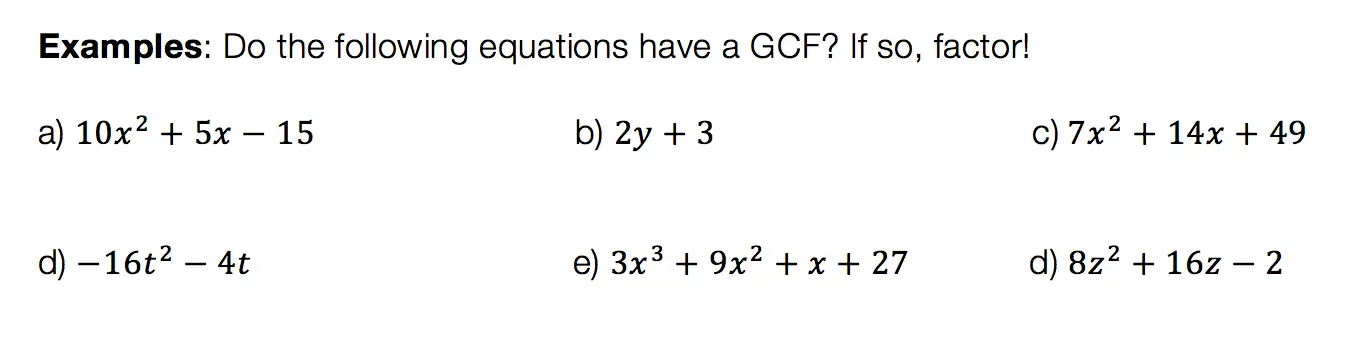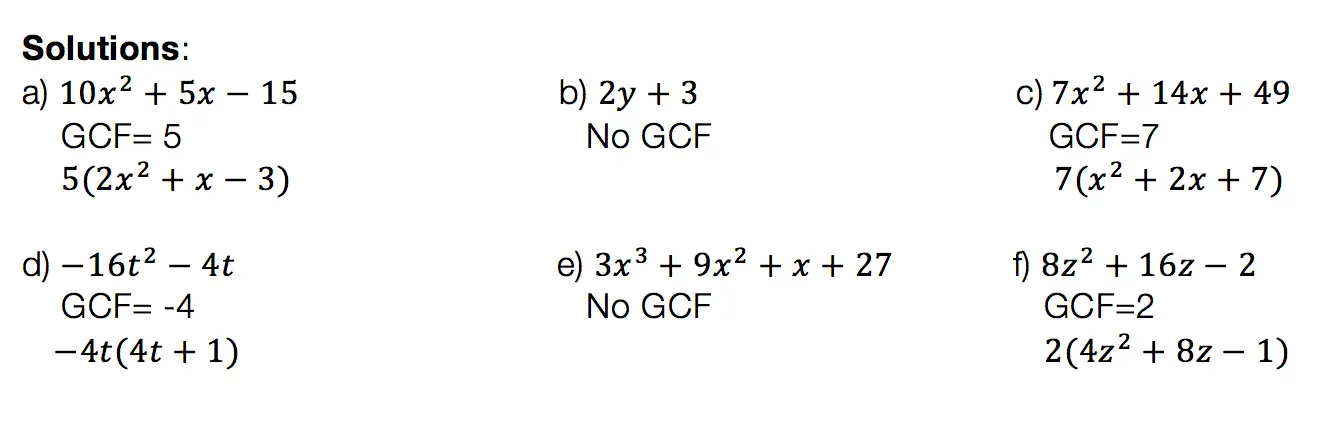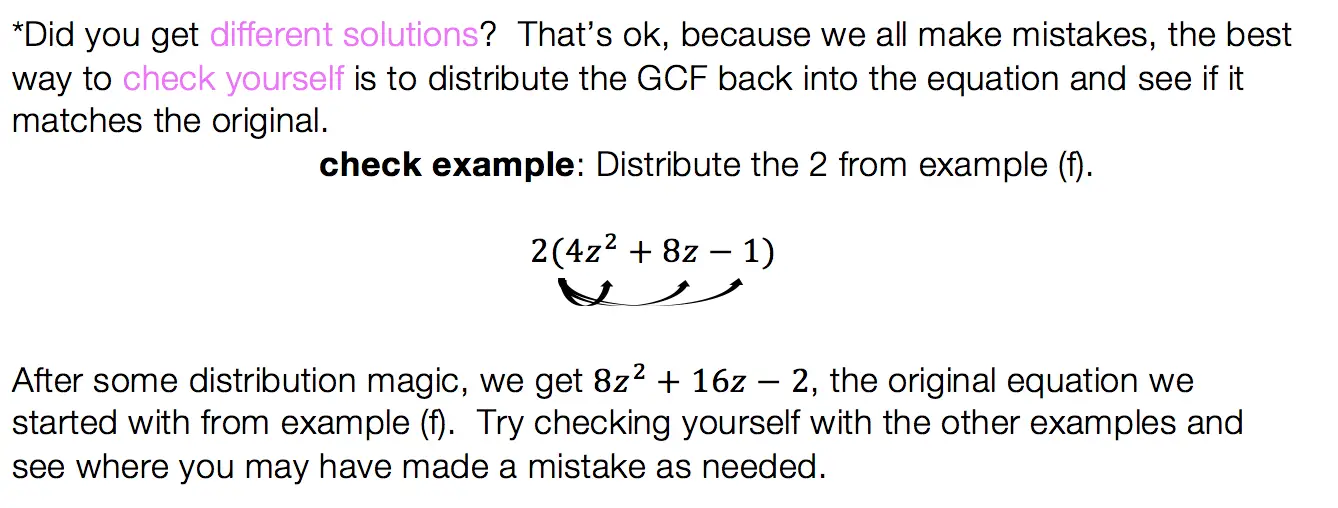## Product/Sum:

This factoring method is for quadratic equations only! That means the equation takes on the following form: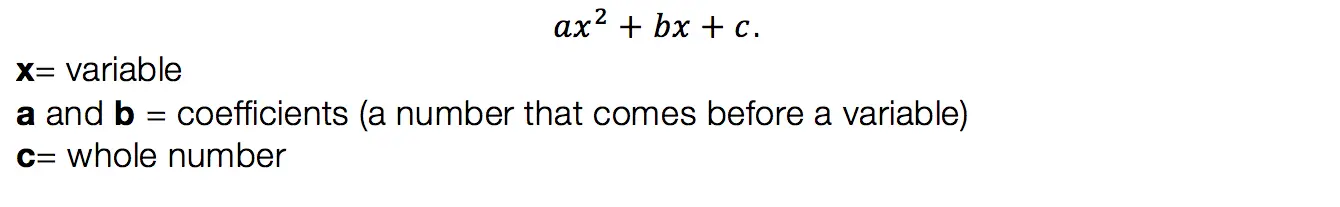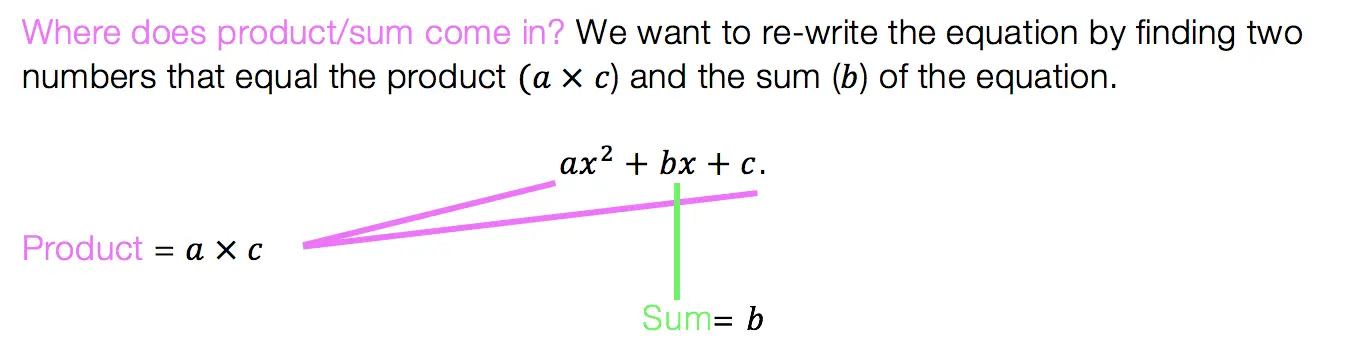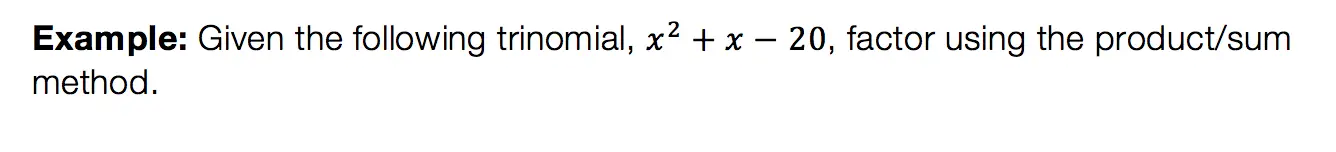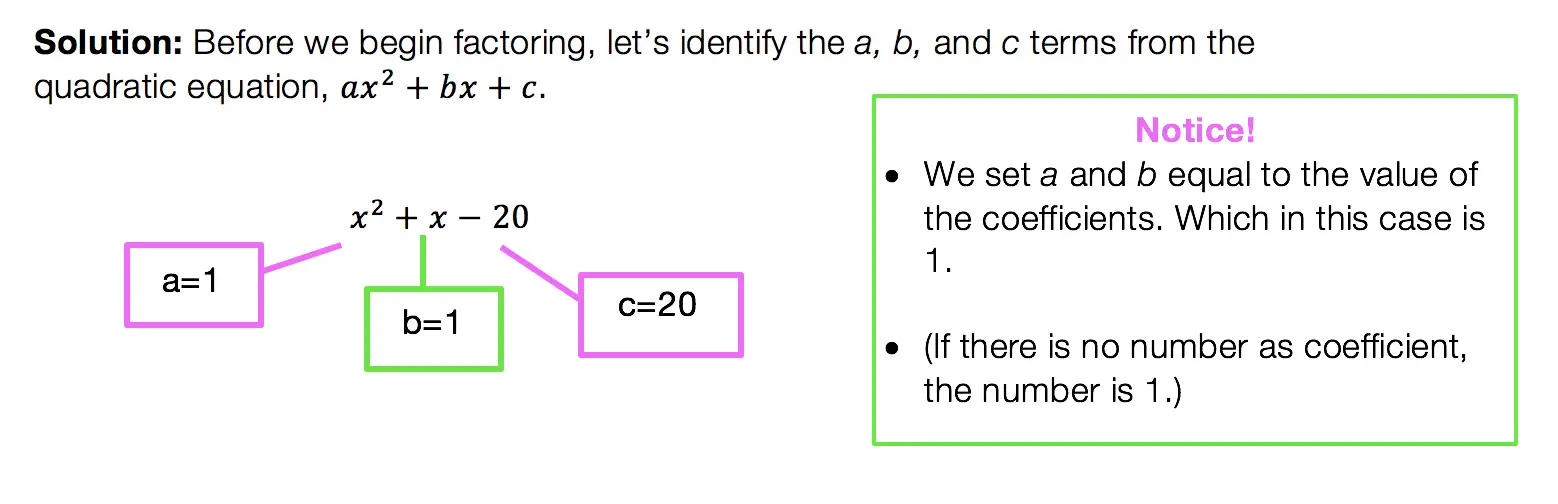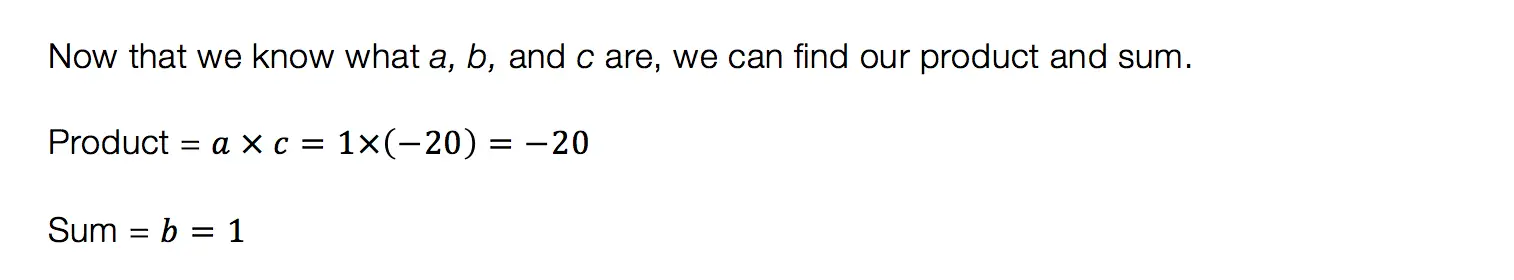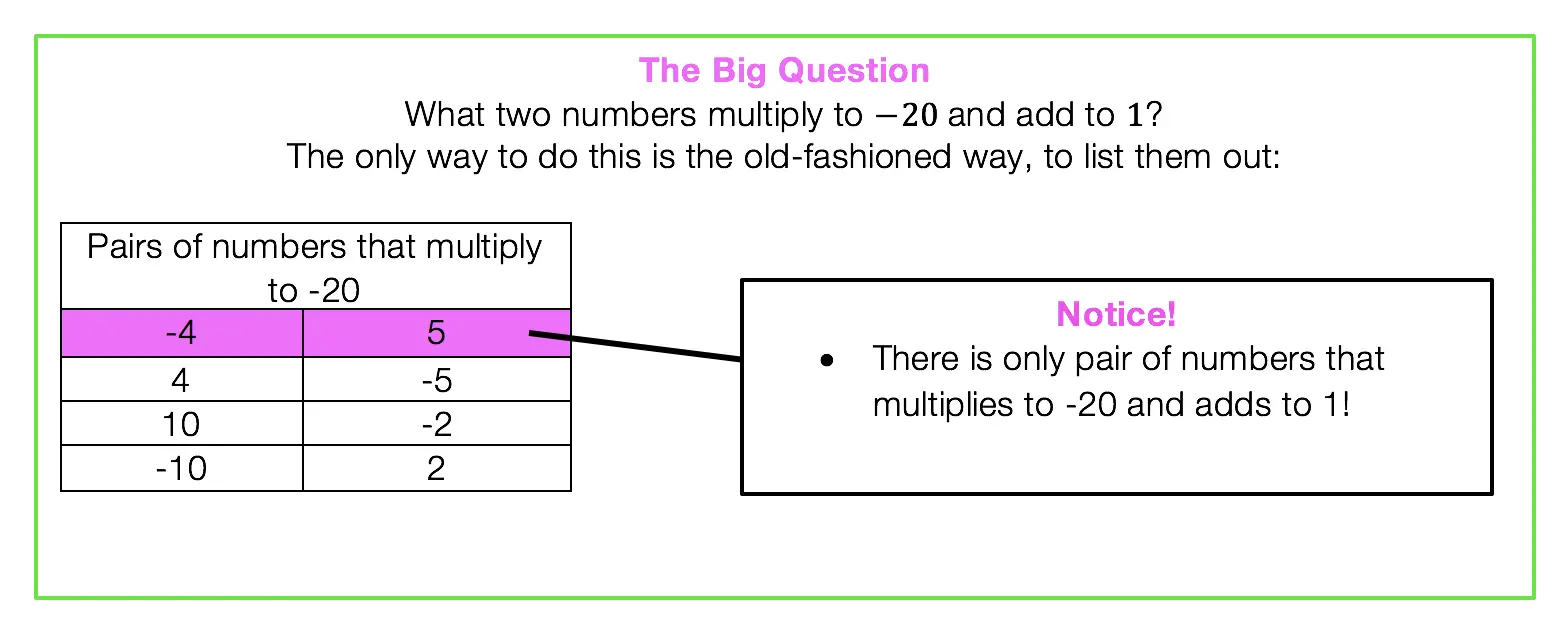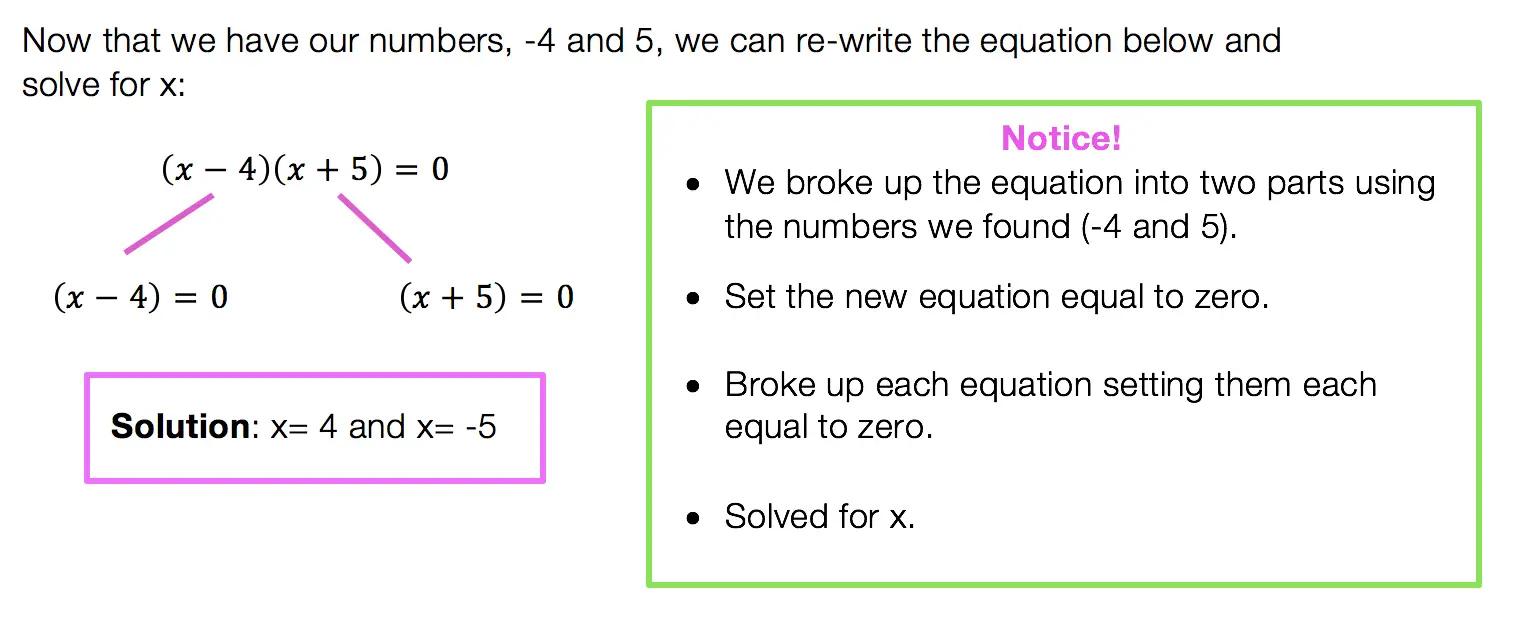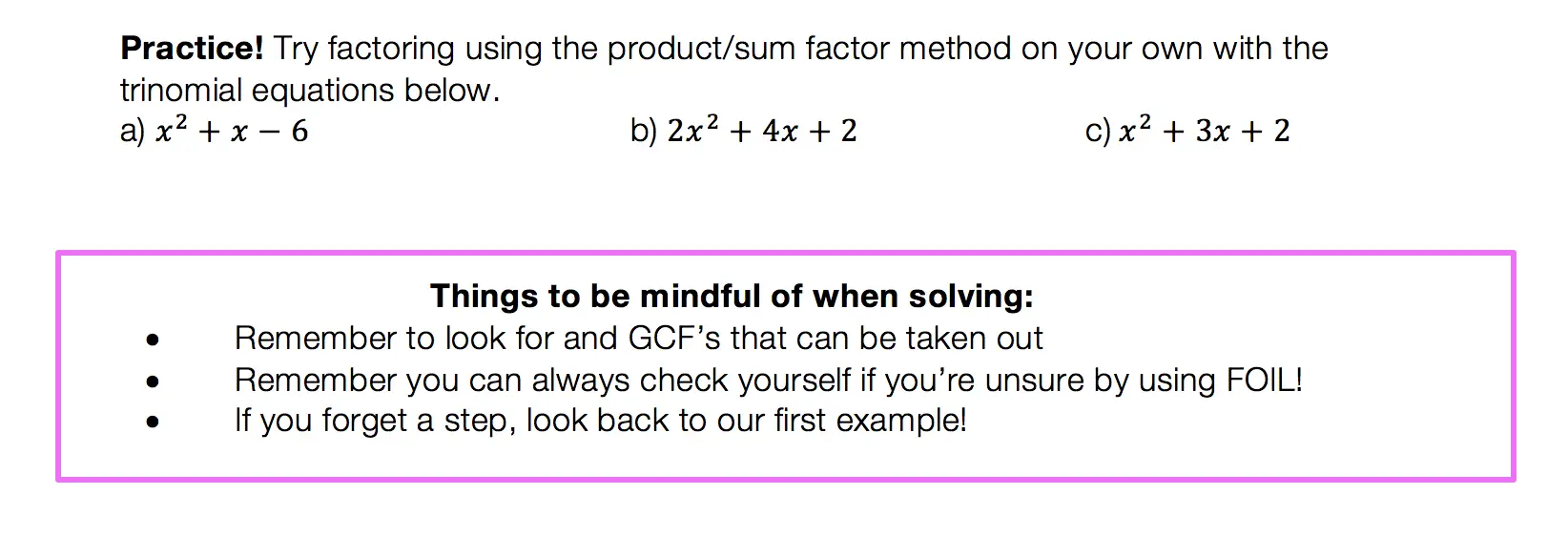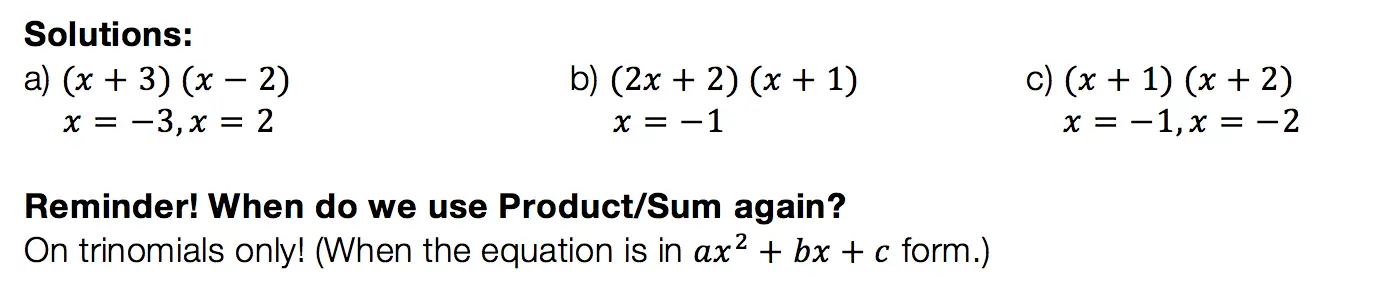## Difference of Two Squares DOTS)

Not to play favorites or anything, but DOTS is the easiest and most lovable of the factoring methods.  This factoring method just makes you feel all warm and fuzzy inside or maybe that’s just me).  Before we get into how to do DOTS, let’s talk about when?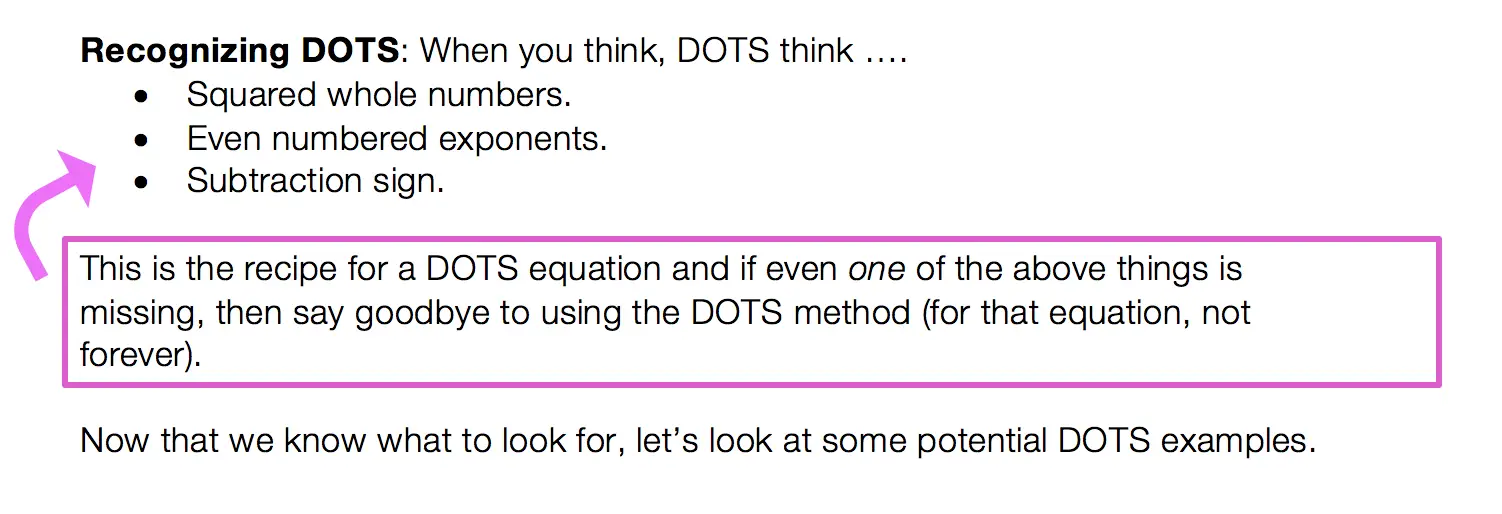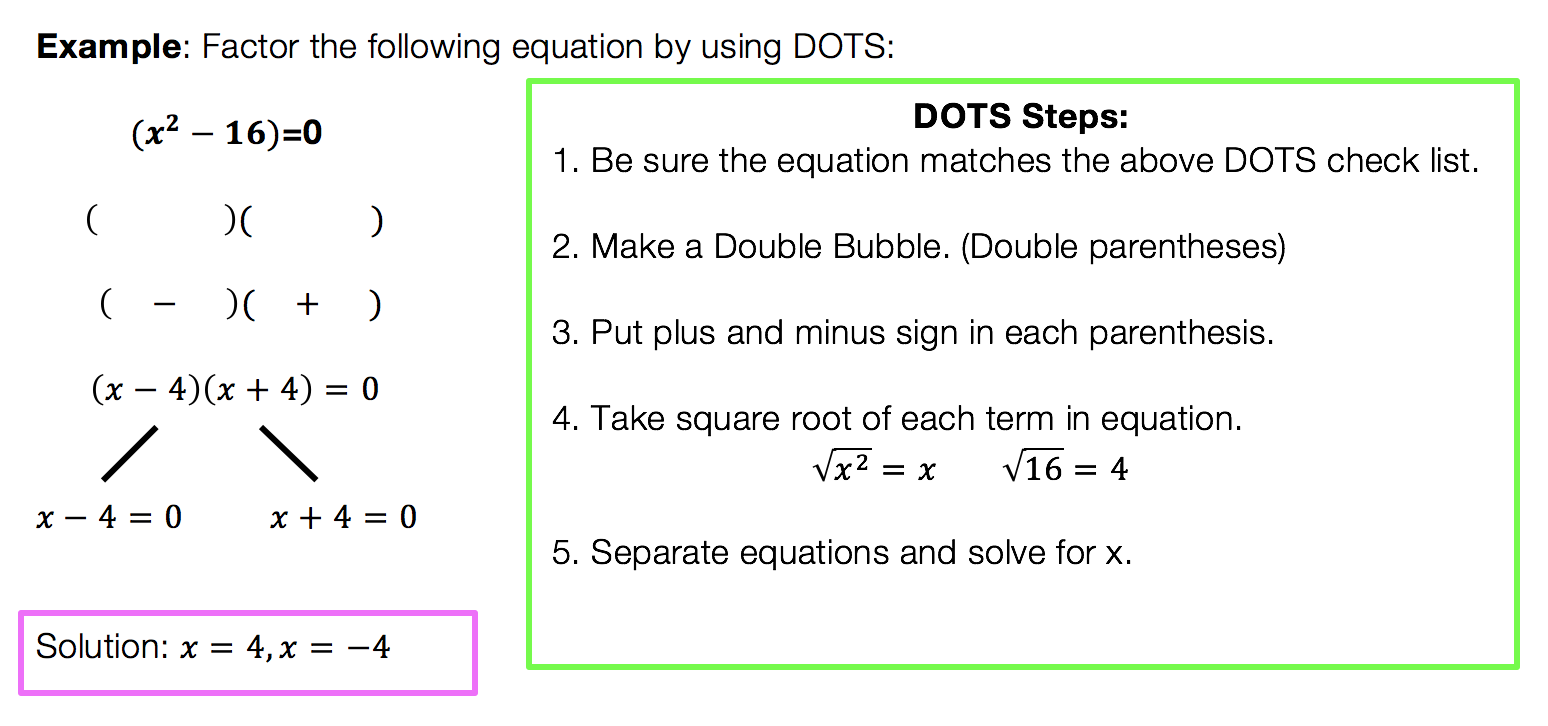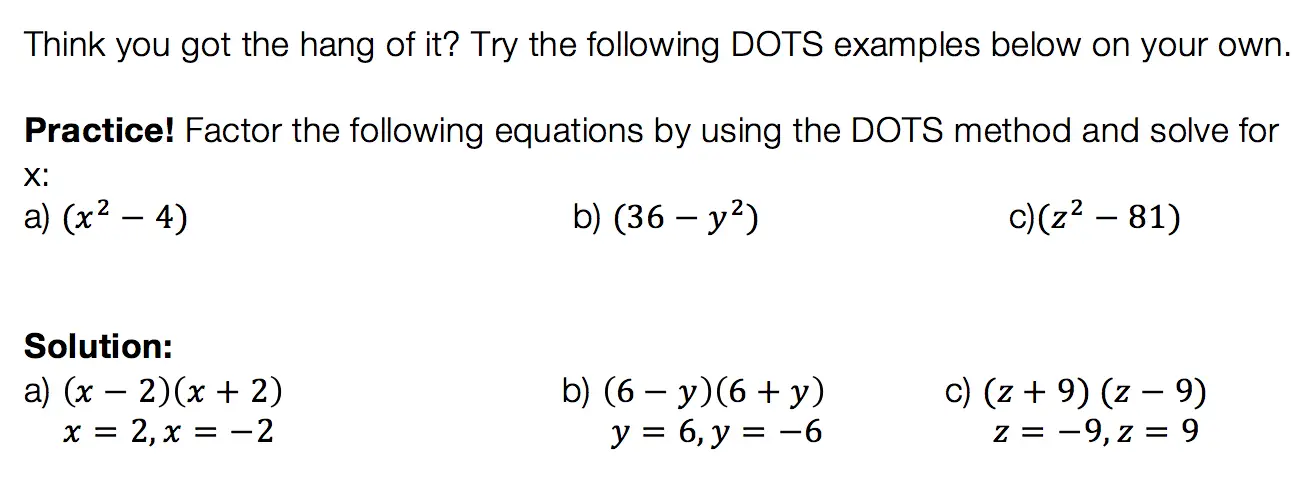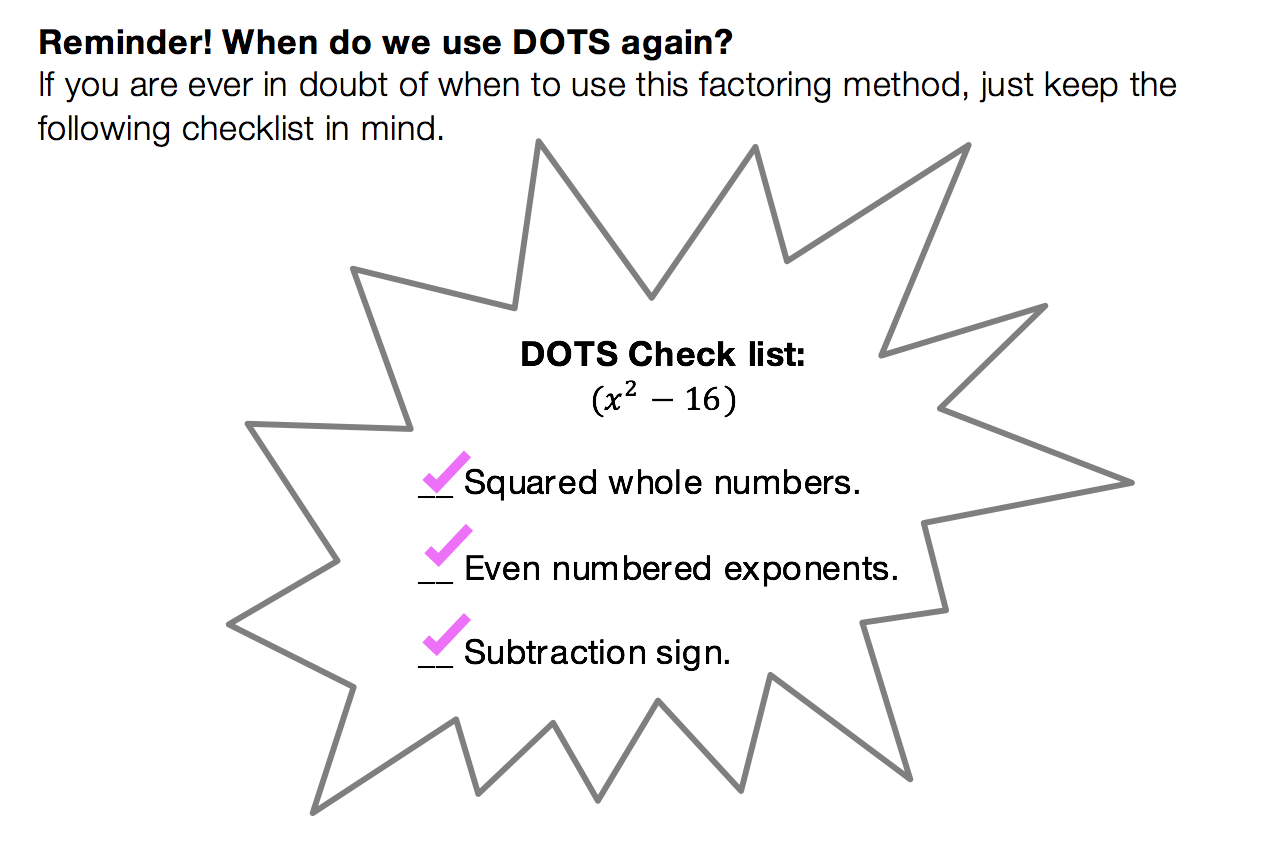We have heard of the quadratic equations, so how id the quadratic formula different?

The Answer: The Quadratic Formula is what we use to factor any trinomial. You can use product/sum on trinomials like we discussed earlier, but this may not always work out easy.  The Quadratic Formila on the other hand will work every time!

Low and behold, the Quadratic Formula: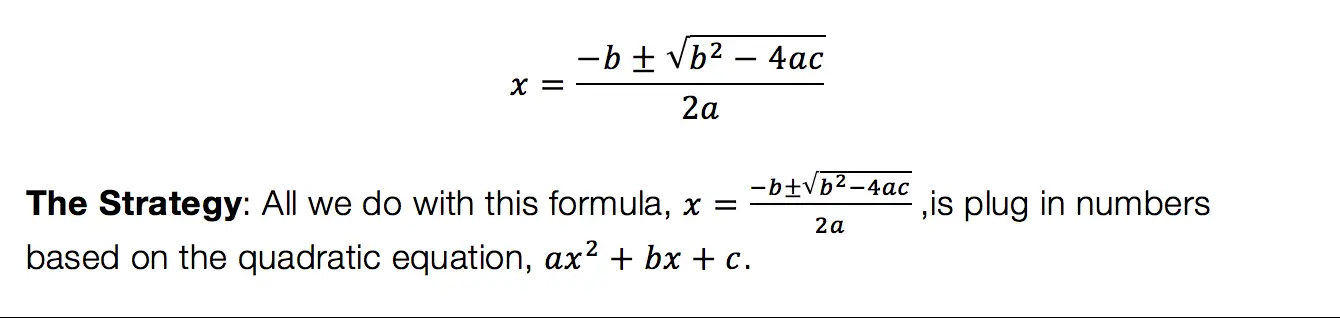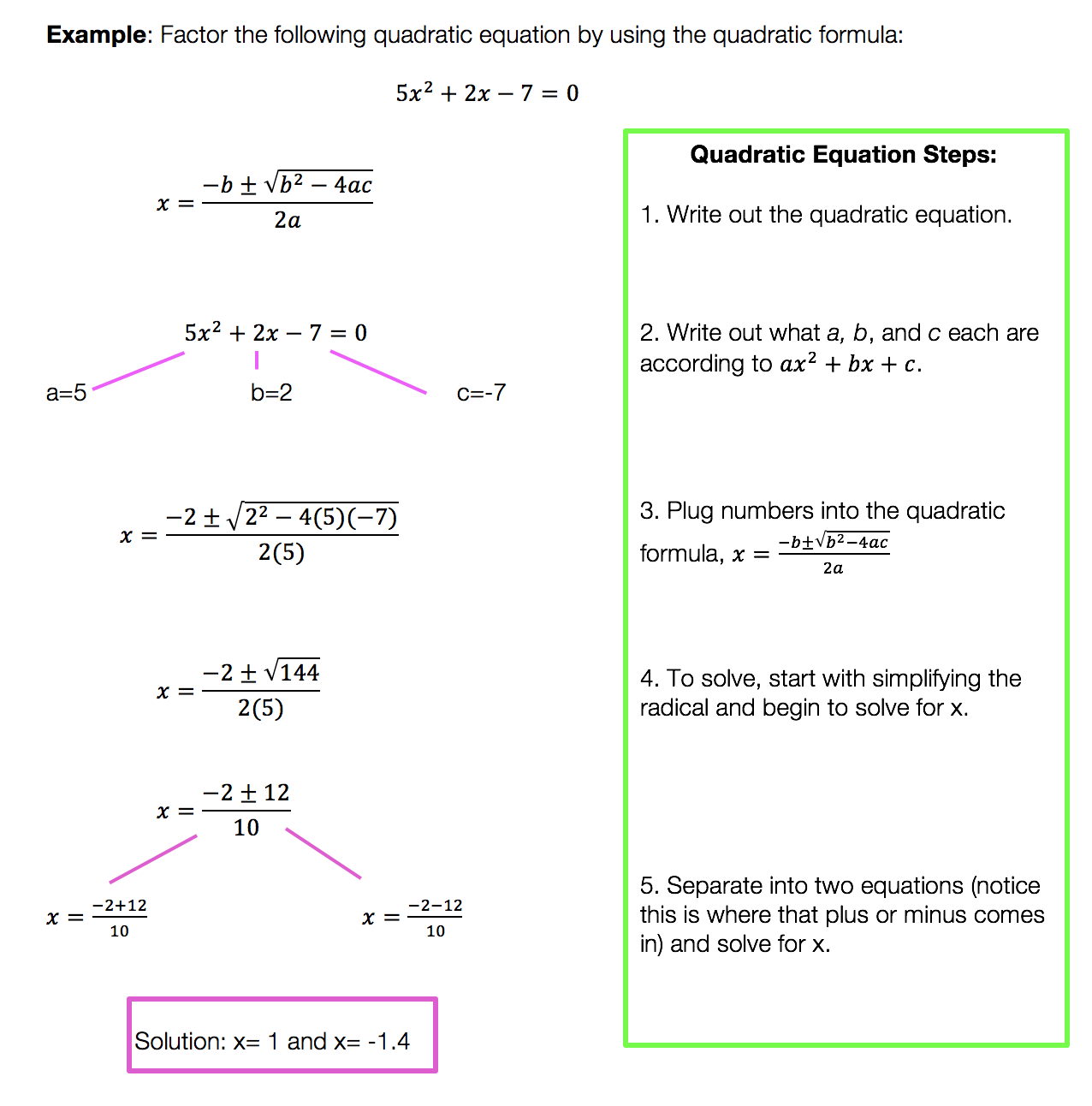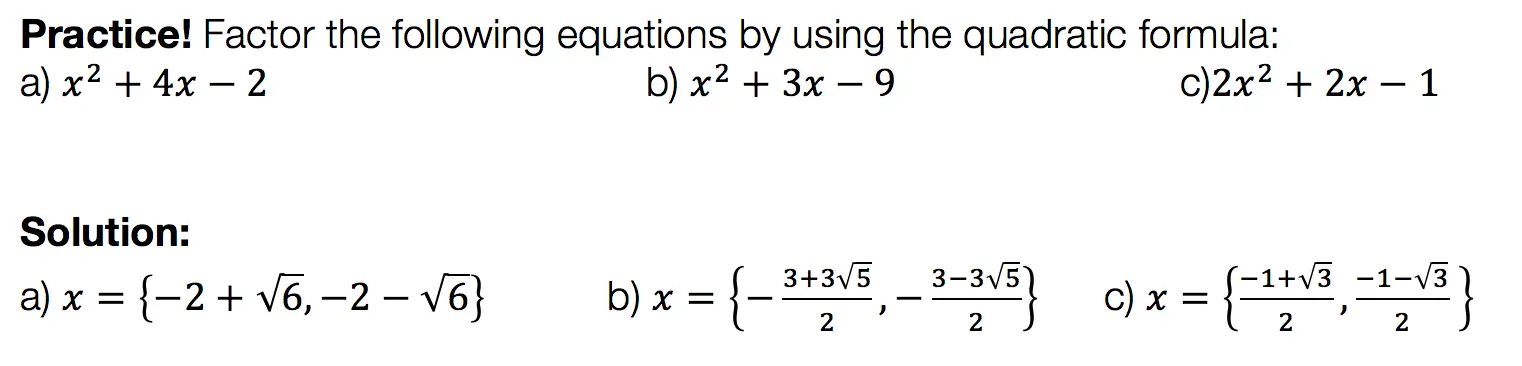Want more Mathsux?  Don’t forget to check out our Youtube channel and more below! And if you have any questions, please don’t hesitate to comment below. Happy Calculating! 🙂

Looking for more on Quadratic Equations and functions? Check out the following Related posts!

Factoring

Factor by Grouping

Completing the Square

The Discriminant

Is it a Function?

Quadratic Equations with 2 Imaginary Solutions

Imaginary and Complex Numbers

Focus and Directrix of a Parabola

## ‘Math Suks’ ~ Jimmy Buffett

Need to relax after studying? Close to wanting to “burn your textbook”? This super chill and somewhat cheesy song may help! Coming to you from Jimmy Buffett, the singer has a relaxed, island/ska like vibe to his music.  The lyrics come close to sounding like an after school special until the words “Math Sucks” are sung over and over again.  Enjoy! 🙂

‘Math Suks’ by Jimmy Buffet Lyrics:

If necessity is the mother of invention
Then I’d like to kill the guy who invented this
The numbers come together in some kind of a third dimension
A regular algebraic bliss.

Any two plus two will never get you five.
There are fractions in my subtraction and x don’t equal y
But my homework is bound to multiply.

Math suks math suks
I’d like to burn this textbook, I hate this stuff so much.
Math suks math suks
Sometimes I think that I don’t know that much
But math suks.

I got so bored with my homework, I turned on the TV.
The beauty contest winners were all smiling through their teeth.
Then they asked the new Miss America
Hey babe can you add up all those bucks?
She looked puzzled, then just said
“Math Suks”.

Math suks math suks
You don’t even have to spell it,
All you have to do is yell it…
Math suks math suks
Sometime times I think that I don’t know that much
But math suks.

Geometry, trigonometry and if that don’t tax your brain
There are numbers to big to be named
Numerical precision is a science with a mission
And I think it’s gonna drive me insane.

Parents fighting with their children, and the Congress can’t agree
Teachers and their students are all jousting constantly.
Management and labor keep rattling old sabers
Quacking like those Peabody ducks.

Math suks math suks
You don’t even have to spell it,
All you have to do is yell it…
Math suks math suks
Sometime times I think that I don’t know that much
But math suks